# Vectors

This is a vector: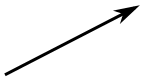A vector has magnitude (size) and direction:The length of the line shows its magnitude and the arrowhead points in the direction.And it doesn't matter which order we add them, we get the same result: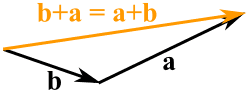### Example: A plane is flying along, pointing North, but there is a wind coming from the North-West.The two vectors (the velocity caused by the propeller, and the velocity of the wind) result in a slightly slower ground speed heading a little East of North.

If you watched the plane from the ground it would seem to be slipping sideways a little.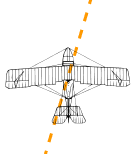Have you ever seen that happen? Maybe you have seen birds struggling against a strong wind that seem to fly sideways. Vectors help explain that.

Velocity, acceleration, force and many other things are vectors.

## Subtracting

We can also subtract one vector from another:

• first we reverse the direction of the vector we want to subtract,
• then add them as usual: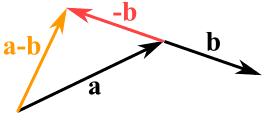ab

## Notation

A vector is often written in bold, like a or b.

 A vector can also be written as the letters of its head and tail with an arrow above it, like this:## Calculations

Now ... how do we do the calculations?

The most common way is to first break up vectors into x and y parts, like this: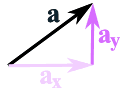The vector a is broken up into
the two vectors ax and ay

(We see later how to do this.)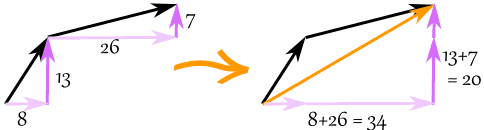The vector (8, 13) and the vector (26, 7) add up to the vector (34, 20)

### Example: add the vectors a = (8, 13) and b = (26, 7)

c = a + b

c = (8, 13) + (26, 7) = (8+26, 13+7) = (34, 20)

When we break up a vector like that, each part is called a component:

## Subtracting Vectors

To subtract, first reverse the vector we want to subtract, then add.

### Example: subtract k = (4, 5) from v = (12, 2)

a = v + −k

a = (12, 2) + −(4, 5) = (12, 2) + (−4, −5) = (12−4, 2−5) = (8, −3)

## Magnitude of a Vector

The magnitude of a vector is shown by two vertical bars on either side of the vector:

|a|

OR it can be written with double vertical bars (so as not to confuse it with absolute value):

||a||

We use Pythagoras' theorem to calculate it:

|a| = √( x2 + y2 )

### Example: what is the magnitude of the vector b = (6, 8) ?

|b| = √( 62 + 82) = √( 36+64) = √100 = 10

A vector with magnitude 1 is called a Unit Vector.

## Vector vs Scalar

A scalar has magnitude (size) only.

Scalar: just a number (like 7 or −0.32) ... definitely not a vector.

A vector has magnitude and direction, and is often written in bold, so we know it is not a scalar:

• so c is a vector, it has magnitude and direction
• but c is just a value, like 3 or 12.4

## Multiplying a Vector by a Scalar

When we multiply a vector by a scalar it is called "scaling" a vector, because we change how big or small the vector is.

### Example: multiply the vector m = (7, 3) by the scalar 3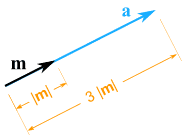a = 3m = (3×7, 3×3) = (21, 9)

It still points in the same direction, but is 3 times longer

(And now you know why numbers are called "scalars", because they "scale" the vector up or down.)

## Multiplying a Vector by a Vector (Dot Product and Cross Product)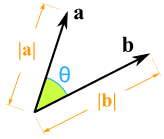How do we multiply two vectors together? There is more than one way! The scalar or Dot Product (the result is a scalar). The vector or Cross Product (the result is a vector). (Read those pages for more details.)

## More Than 2 Dimensions

Vectors also work perfectly well in 3 or more dimensions:The vector (1, 4, 5)

### Example: add the vectors a = (3, 7, 4) and b = (2, 9, 11)

c = a + b

c = (3, 7, 4) + (2, 9, 11) = (3+2, 7+9, 4+11) = (5, 16, 15)

### Example: what is the magnitude of the vector w = (1, −2, 3) ?

|w| = √( 12 + (−2)2 + 32 ) = √( 1+4+9) = √14

Here is an example with 4 dimensions (but it is hard to draw!):

### Example: subtract (1, 2, 3, 4) from (3, 3, 3, 3)

(3, 3, 3, 3) + −(1, 2, 3, 4)
= (3, 3, 3, 3) + (−1,−2,−3,−4)
= (3−1, 3−2, 3−3, 3−4)
= (2, 1, 0, −1)

## Magnitude and Direction

We may know a vector's magnitude and direction, but want its x and y lengths (or vice versa):<=>Vector a in Polar Coordinates Vector a in Cartesian Coordinates

You can read how to convert them at Polar and Cartesian Coordinates, but here is a quick summary:

From Polar Coordinates (r,θ) From Cartesian Coordinates (x,y) to Cartesian Coordinates (x,y) to Polar Coordinates (r,θ) x = r × cos( θ ) y = r × sin( θ ) r = √ ( x2 + y2 ) θ = tan-1 ( y / x )## An Example

Sam and Alex are pulling a box.

• Sam pulls with 200 Newtons of force at 60°
• Alex pulls with 120 Newtons of force at 45° as shown

What is the combined force, and its direction?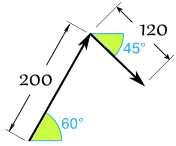First convert from polar to Cartesian (to 2 decimals):

Sam's Vector:

• x = r × cos( θ ) = 200 × cos(60°) = 200 × 0.5 = 100
• y = r × sin( θ ) = 200 × sin(60°) = 200 × 0.8660 = 173.21

Alex's Vector:

• x = r × cos( θ ) = 120 × cos(−45°) = 120 × 0.7071 = 84.85
• y = r × sin( θ ) = 120 × sin(−45°) = 120 × -0.7071 = −84.85

Now we have: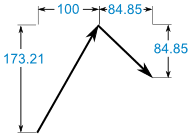(100, 173.21) + (84.85, −84.85) = (184.85, 88.36)

That answer is valid, but let's convert back to polar as the question was in polar:

• r = √ ( x2 + y2 ) = √ ( 184.852 + 88.362 ) = 204.88
• θ = tan-1 ( y / x ) = tan-1 ( 88.36 / 184.85 ) = 25.5°

And we have this (rounded) result: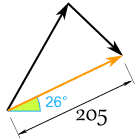And it looks like this for Sam and Alex:They might get a better result if they were shoulder-to-shoulder!